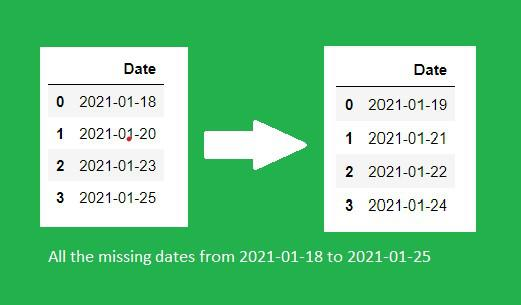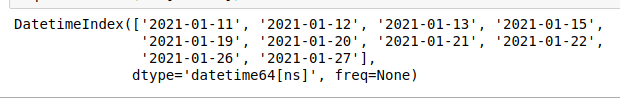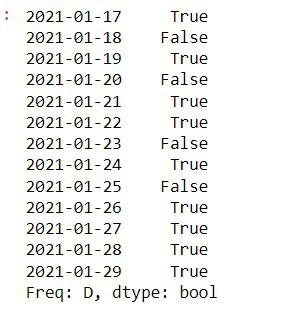# Check missing dates in Pandas

• Last Updated : 15 Sep, 2022

In this article, we will learn how to check missing dates in Pandas.

A data frame is created from a dictionary of lists using pd.DataFrame() which accepts the data as its parameter. Note that here, the dictionary consists of two lists named Date and Name. Both of them are of the same length and some dates are missing from the given sequence of dates ( From  2021-01-18 to 2021-01-25 ).Check missing dates

## Checking whether the given Date is missing from the data frame

Here we are returning True if the date is present and False if the date is missing from the data frame.

## Python3

 `import` `pandas as pd` `# A dataframe from a dictionary of lists` `data ``=` `{``'Date'``: [``'2021-01-18'``, ``'2021-01-20'``,` `                 ``'2021-01-23'``, ``'2021-01-25'``],` `       ``'Name'``: [``'Jia'``, ``'Tanya'``, ``'Rohan'``, ``'Sam'``]}`   `df ``=` `pd.DataFrame(data)`   `df[``'Date'``] ``=` `pd.to_datetime(df[``'Date'``])`   `d``=``'2021-01-19'` `print``(pd.to_datetime(d) ``in` `df[``'Date'``].tolist())`

Output:

`True`

## Using data_range() and .difference() function to check missing dates

Example 1:

df.set_index() method sets the dates as the index for the data frame we created.  One can simply print the data frame using print(df) to see it before and after setting the Date as an index. Now, once we have set the date as the index, we convert the given list of dates into a DateTime object. Originally, the dates in our list are strings that need to be converted into the DateTime object. Pandas provide us with a method called to_datetime() which converts the date and time in string format to a DateTime object.

## Python3

 `#import pandas` `import` `pandas as pd`   `# A dataframe from a dictionary of lists` `data ``=` `{``'Date'``: [``'2021-01-18'``, ``'2021-01-20'``, ` `                 ``'2021-01-23'``, ``'2021-01-25'``],` `        ``'Name'``: [``'Jia'``, ``'Tanya'``, ``'Rohan'``, ``'Sam'``]}` `df ``=` `pd.DataFrame(data)`   `# Setting the Date values as index` `df ``=` `df.set_index(``'Date'``)`   `# to_datetime() method converts string ` `# format to a DateTime object` `df.index ``=` `pd.to_datetime(df.index)`   `# dates which are not in the sequence ` `# are returned` `print``(pd.date_range(` `  ``start``=``"2021-01-18"``, end``=``"2021-01-25"``).difference(df.index))`

Output:

Finally, we get all the dates that are missing between 2021-01-18 and 2021-01-25.

DatetimeIndex([‘2021-01-19’, ‘2021-01-21’, ‘2021-01-22’, ‘2021-01-24′], dtype=’datetime64[ns]’, freq=None)

Pandas.Index.difference() returns a new Index with elements of index not in others. Therefore, by using pd.date_range(start date, end date).difference(Date), we get all the dates that are not present in our list of Dates. The data type returned is an Immutable ndarray-like of datetime64 data.

Example 2:

Let us consider another example. However, this time we will not set the date as an index and will assign freq=’B’ (Business Day Frequency) inside the pd.date_range() function.

Just like the previous example, we make a dataframe from the dictionary of lists. However, this time we do not set the date values as index. Instead, we set the column ‘Total People’ as our index values. Using pd.date_range() function, which takes start date, end date and frequency as parameters, we provide the values. We set the freq= ‘B’  (Business Day Frequency) in order to omit weekends. Finally, Pandas.Index.difference()  takes the Date column as a parameter and returns all those values which are not in the given set of values.

## Python3

 `#import pandas` `import` `pandas as pd`   `# A dataframe from a dictionary of lists` `d ``=` `{``'Date'``: [``'2021-01-10'``, ``'2021-01-14'``, ``'2021-01-18'``, ` `              ``'2021-01-25'``, ``'2021-01-28'``, ``'2021-01-29'``],` `     ``'Total People'``: [``20``, ``21``, ``19``, ``18``, ``13``, ``56``]}` `df ``=` `pd.DataFrame(d)`   `# Setting the Total People as index` `df ``=` `df.set_index(``'Total People'``)`   `# to_datetime() method converts string ` `# format to a DateTime object` `df[``'Date'``] ``=` `pd.to_datetime(df[``'Date'``])`   `# dates which are not in the sequence ` `# are returned` `my_range ``=` `pd.date_range(` `  ``start``=``"2021-01-10"``, end``=``"2021-01-31"``, freq``=``'B'``)`   `print``(my_range.difference(df[``'Date'``]))`

Output:Check missing dates

Note that all the missing values except 2021-01-23, 2021-01-24, and 2021-01-30 are returned because we have set freq=’B’ which omits all the weekends.

## Using reindex() function to check missing dates

Here we are typecasting the string type date into datetime type and with help of reindex() we are checking all the dates that are missing in the given data Frame and assign it to True otherwise assign it to False.

## Python3

 `import` `pandas as pd` ` `  `# A dataframe from a dictionary of lists` `data ``=` `{``'Date'``: [``'2021-01-18'``, ``'2021-01-20'``,` `                 ``'2021-01-23'``, ``'2021-01-25'``],` `       ``'Name'``: [``'Jia'``, ``'Tanya'``, ``'Rohan'``, ``'Sam'``]}` `df ``=` `pd.DataFrame(data)`   `df[``'Date'``] ``=` `pd.to_datetime(df[``'Date'``])`   `df.set_index(``'Date'``, inplace``=``True``)`   `df.reindex(pd.date_range(``'2021-01-17'``, ``'2021-01-29'``)` `                        ``).isnull().``all``(``1``)`

Output:Check missing dates

My Personal Notes arrow_drop_up
Recommended Articles
Page :